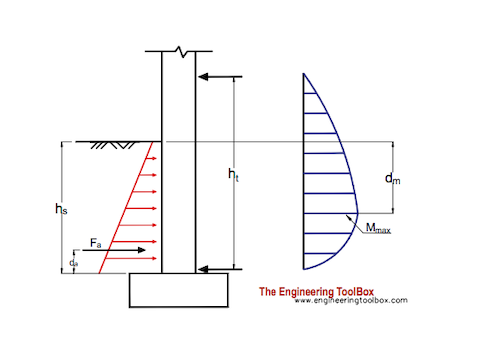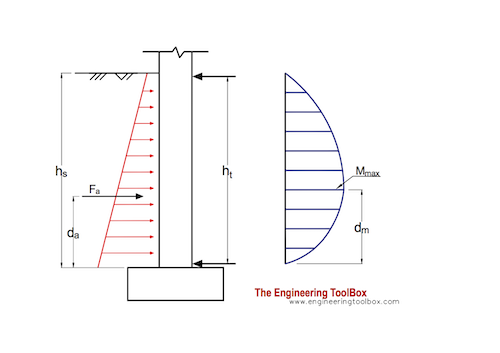Engineering ToolBox - Resources, Tools and Basic Information for Engineering and Design of Technical Applications!

# Earth Pressure Acting on Basement Walls

## Calculate lateral earth pressure acting on basement walls.

### 1st Floor above Ground Level

The forces that keeps the basement wall in position - the force at the basement floor/slab and the force from the 1. floor joists above ground level - are indicated in the figure below.The resultant force due to the earth pressure acting on a basement wall can be calculated as

Fa = 0.5 K γ hs2                             (1a)

where

Fa = resultant force acting on the basement wall (kN)

hs = height of backfill soil (m)

γ = specific weight of backfill soil (kN/m3)

K = coefficient of earth pressure at rest

Coefficient of earth pressure at rest can be calculated as

K = μ / (1 - μ)                      (1b)

where

μ = Poisson's ratio

Poisson's ratios for some typical backfill soils:

• clay: 0.41
• sand : 0.29
• sandy clay: 0.37
• sandy loam: 0.31

Specific weight can be calculated as

γ = ρ ag                               (1c)

where

ρ = density of soil (kg/m3)

ag = acceleration of gravity (9.81 m/s2)

Density for some typical backfill materials:

• clay, dry: 1600 kg/m3
• sand, dry: 1555 kg/m3

The acting position of the acting force can be calculated as

da = hs / 3                           (1d)

where

da = distance from the bottom of the basement wall    (m)

The maximum bending moment acting in the basement wall can be calculated as

Mmax = (Fa hs / 3 ht) (hs + (2 hs / 3)(hs / (3 ht))1/2)                            (1e)

where

Mmax = maximum moment in the basement wall (Nm)

The position of the max moment in the basement wall can be calculated as

dm = hs (hs / (3 ht))1/2                 (1f)

Note! - cracking of a basement wall is likely to occur where the moment is at the maximum. Due to tension reinforcement bars should be concentrated closer to the inside wall.

### 1st Floor at Ground Level

The forces that keeps the basement wall in position - the force at the basement floor/slab and the force from the 1. floor joists at ground level - are indicated in the figure below.The resultant force due to the earth pressure acting on a basement wall can be calculated as

Fa = 0.5 K γ hs2                             (2a)

The acting position of the acting force can be calculated as

da = hs / 3                           (2b)

where

da = distance from the bottom of the basement wall    (m)

The maximum moment acting in the basement wall can be calculated as

Mmax = 0.128 Fa hs                                (2c)

where

Mmax = maximum moment in the basement wall (Nm)

The position of the max. moment in the basement wall can be calculated as

dm = 0.42 hs                   (2e)

#### Example - Earth Pressure on Basement Wall

A basement wall with height 2.5 m is backfilled with sand.

The Poisson's ratio for sand is 0.29 and the coefficient of earth pressure can be calculated as

K = 0.29 / (1 - 0.29)

= 0.41

The specific weight for the sand can be calculated as

γ = (1555 kg/m3) (9.81 m/s2)

= 15255 N/m3

= 15.3 kN/m3

The resultant force acting on the basement wall can be calculated as

Fa = 0.5 (0.41) (15.3 kN/m3) (2.5 m)2

= 19.6 kN

The acting position of the resultant force can be calculated as

da = (2.5 m) / 3

= 0.83 m

The maximum moment acting in the basement wall can be calculated as

Mmax = 0.128 (19.6 kN) (2.5 m)

= 6.3 kNm

The position of the max. moment in the basement wall can be calculated as

dm = 0.42 (2.5 m)

= 1.05

## Related Topics

• ### Statics

Loads - forces and torque, beams and columns.

## Related Documents

• ### Concrete Footings - Volume

Estimate required concrete footing volume.
• ### Concrete Mixtures

Cement, sand and gravel mixtures.
• ### Concrete Properties

Properties of normal strength Portland cement concrete.
• ### Concrete Slabs - Required Thickness

Required thickness of concrete slabs.
• ### Concrete Slabs - Volume Estimates

Estimate required concrete volume per sq. ft.
• ### Concrete Walls - Volume

Estimate concrete wall volume.
• ### Dirt and Mud - Densities

Densities - pound per cubic foot and kilograms per cubic metre - of dirt and mud.
• ### Rebar - Metric Reinforcing Bar

Reinforcing bar - European metric dimensions.

• ### Soil - Earth Weight and Composition

Typical weight and composition of soil.

## Engineering ToolBox - SketchUp Extension - Online 3D modeling!

Add standard and customized parametric components - like flange beams, lumbers, piping, stairs and more - to your Sketchup model with the Engineering ToolBox - SketchUp Extension - enabled for use with older versions of the amazing SketchUp Make and the newer "up to date" SketchUp Pro . Add the Engineering ToolBox extension to your SketchUp Make/Pro from the Extension Warehouse !

We don't collect information from our users. More about

## Citation

• The Engineering ToolBox (2003). Earth Pressure Acting on Basement Walls. [online] Available at: https://www.engineeringtoolbox.com/basement-wall-earth-pressure-d_558.html [Accessed Day Month Year].

Modify the access date according your visit.

9.19.12